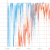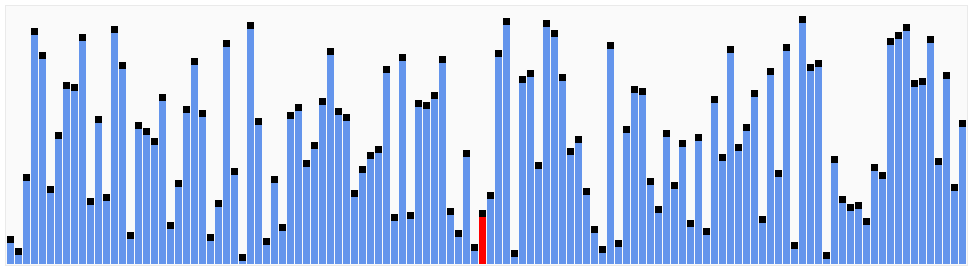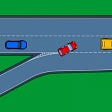# Abstract Data Types and Data Structures

In this post, we are going to explore what ADTs and data structures are and how they are different from each other.

Among various ADTs, the main topics to be discussed here are:

• Array
• List
• Stack
• Queue

Let’s first discuss those as ADTs and how to implement them as data structures.

# Abstract Data Types

It’s a way of classifying data structures according to their behavior.

## Stack

A stack is a type of list that you should insert elements in a specific order. It is alternatively called as LIFO (Last In, First Out). You can think of it as a vertical cylinder. The last thing you put is taken out first.

Basic operations include

• push(item)
• pop()
• isEmpty()

## Queue

A queue is also a list like a stack, but it’s called FIFO (First In, First Out). The first thing to be put is taken out first. You can imagine a vertical cylinder that has the exit at the bottom, the entry at the top.

To compare those two:

• Stack has only one entry/exit at the top.
• The queue has two holes: entry at the top, exit at the bottom.

Basic operations include

• enqueue(item)
• dequeue()

# Data Structures

They represent how you can actually implement ADTs. There are appropriate ways to implement each ADTs like the following:

• Array: Array
• Stack: Array & Linked List
• Queue: Array & Linked List
• Set: Hash Table
• Map: Hash Table
• Priority Queue: Heap

## Array

The array is a linear data structure that stores homogeneous elements.

The memory address of the ith element is b + (i * size) where b is the base address (the memory address of the first element.)

Both the time complexity of getting and setting the element is O(1) since we can easily compute the memory address of each element using the equation above.

## Stack

For stacks, the time complexity of pushing and popping the element is also O(1). Remember that a stack is also called “LIFO.” Let’s say there are n elements. Then, push() adds the element to the memory address of (b + n * size) and pop() returns the element on that address.

What if we try to push an element and the pointer exceeds the stack bound? We get the famous stack overflow! The size of the stack is determined by the amount of available memory, machine architecture, programming language, and so on.

A. Resizing stacks

But what if we want to push an element when the stack is full? I referred to this awesome blog by Emory Oxford College and it introduces a creative workaround that reduces the time complexity of O(N²) to O(N).

There are two main notions to keep in mind:

1. Grow: Double the size of the array
2. Shrink: Halve the size of the array

A-1. Scenario 1

Let’s say that we want to grow the array when its size reaches an arbitrary number of N. Since the memory for the array was already allocated, we can’t magically append more memories to the end of it. This forces us to create a new stack with one more cell. We have to “read” N elements in the original array and “write” those N elements to the new array.

So, the number of copies we have to make is

Why not just N though? When copying the array, we works like the following way

1. Copy the first element and

## Queue

Can we also implement the queue with an array? While it’s possible, it might not be the best way to do this.

First, initialize an empty array of size N. The head (read) and tail (write) is pointing to one end of the array (to the far right in this example).

Enqueue: Move the tail to the left by the size of the element.

Dequeue: Read the value to which the head is pointing and move the head left by the size of the element.

As you can see, the time complexity of both operations is O(1).

However, this is only the best case. What if the tail is already pointing to the far left and we want to push a new element?

We will have to copy all the elements and move them to the far right if there’s enough space. The time complexity is, therefore, O(N).

We should raise questions at this point. Can we address this scenario without having to copy all the elements?

This gives rise to using a circular array with a modular operation.

I’ve implemented this in Python for fun:

`class Queue:    def __init__(self, capacity):        self.capacity = capacity        self.queue = [None] * capacity        self.head = 0  # read        self.tail = 0  # write        self.count = 0            def is_empty(self):        return self.count == 0        def is_full(self):        return self.count == self.capacity            def enqueue(self, value):        tail = (self.tail + 1) % self.capacity        if self.is_full():            print("Queue is full")        else:            self.queue[self.tail] = value            self.tail = tail            self.count += 1        print(self.queue)        def dequeue(self):        if self.is_empty():            print("Queue is empty")        else:            print(self.queue[self.head])            self.queue[self.head] = None            self.head = (self.head + 1) % self.capacity            self.count -= 1        print(self.queue)`

--

--

--

Hello, world.

## Unity camera plugin save image data as bytes## Covid-19 brings us closer than ever## GitHub Notifications is their most underrated feature## Speeding up Docker builds in CI## Understanding application routing in Istio## 「Build on Darwinia 2–3」Deploying UNISWAP V2 to Crab/Pangolin Network — I## Containers Save the Day!## Quick Sort## Merge k Sorted Lists using minheap in O(N logK) time## Leetcode Q313. Super Ugly Number (Q266)